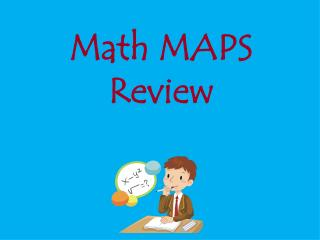DownloadDownload PresentationMath MAPS Review

# Math MAPS Review

Download Presentation## Math MAPS Review

- - - - - - - - - - - - - - - - - - - - - - - - - - - E N D - - - - - - - - - - - - - - - - - - - - - - - - - - -
##### Presentation Transcript

1. Math MAPS Review

2. Numbers & Operations in Base Ten

3. What is the value of the red digit? 146, 521 400 40,000 4,000 4

4. Estimate the sum below. 62 + 27 = 100 80 90 70

5. Estimate the difference below. 469 – 115 = 400 300 200 500

6. Which is the correct way to write 31,725 in expanded form? a. 30,000 + 1,000 + 700 + 20 + 5 = 31,725 b. 31,000 + 720 + 5 = 31,725 c. 300,000 + 7000 + 20 + 5= 31,725 d. 30,000 + 1,000 + 700 + 5 = 31, 725

7. 143 x 2 = 145 285 186 286

8. Identify the number shown below. 437 4,370 4,037 4,307

9. Numbers & Operations- Fractions

10. Identify the fraction of the circle that is green. c. a. b. d.

11. Identify the model below that shows shaded yellow. c. a. b.

12. Which of the following models shows a. c. d. b.

13. What fraction of the circles are gray? ¼ c. 1/6 1/5 d. 1/7

14. What fraction is 3/6 equivalent to? 1/8 c. 1/6 ½ d. ¼

15. Anna and Tom ordered a pizza. Anna ate ¼ of the pizza and Tom at 2/4 of the pizza. How much of the pizza did Anna and Tom eat altogether? 3/8 ¼ 1/3 3/4

16. Mary baked a cake. She ate 3/8 of the cake and gave the rest away. How much cake did she give away? 5/8 2/8 3/8 4/8

17. Paul bought a candy bar for his friends. He divided the candy bar equally between 3 friends. What fraction of the candy bar did each friend get? 3/3 ¾ 1/3 3/1

18. Operations & Algebraic Thinking